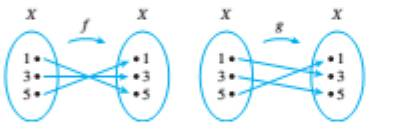Chapter 7.3, Problem 1ES### Discrete Mathematics With Applicat...

5th Edition
EPP + 1 other
ISBN: 9781337694193

#### Solutions

Chapter
Section### Discrete Mathematics With Applicat...

5th Edition
EPP + 1 other
ISBN: 9781337694193
Textbook Problem
1 views

# In each of 1 and 2, functions f and g are defined by arrow diagrams. Find g ∘ f and f ∘ g and determine g ∘ f equals f ∘ g .To determine

To find:

fg,gf and check also whether g o f equals f o g.

Explanation

Given information:

Concept used:

g o f=g(f(x))f o g=f(g(x))

Calculation:

fg(1)=f{g(1)}=f(3)=3fg(3)=f{g(3)}=f(5)=1fg(5)=f{g(5)}=f(1)=5

Therefore, the function fg can be given as shown below

### Still sussing out bartleby?

Check out a sample textbook solution.

See a sample solution

#### The Solution to Your Study Problems

Bartleby provides explanations to thousands of textbook problems written by our experts, many with advanced degrees!

Get Started